# The SURVEYFREQ Procedure

#### Confidence Limits for Totals

If you specify the CLWT option in the TABLES statement, PROC SURVEYFREQ computes confidence limits for the weighted frequencies (totals) in the crosstabulation tables.

For the total in table cell (r, c), the confidence limits are computed as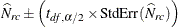where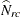is the estimate of the total frequency in table cell (r, c),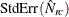is the standard error of the estimate, and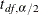is the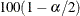percentile of the t distribution with df degrees of freedom calculated as described in the section Degrees of Freedom. The confidence levelis determined by the value of the ALPHA= option, which by default equals 0.05 and produces 95% confidence limits.

The confidence limits for row totals, column totals, and the overall total are computed similarly to the confidence limits for table cell totals.

For each table request, PROC SURVEYFREQ produces a nondisplayed ODS table, Table Summary, which contains the number of observations, strata, and clusters that are included in the analysis of the requested table. When you request confidence limits, the Table Summary data set also contains the degrees of freedom df and the value ofthat is used to compute the confidence limits. See Example 90.3 for more information about this output data set.